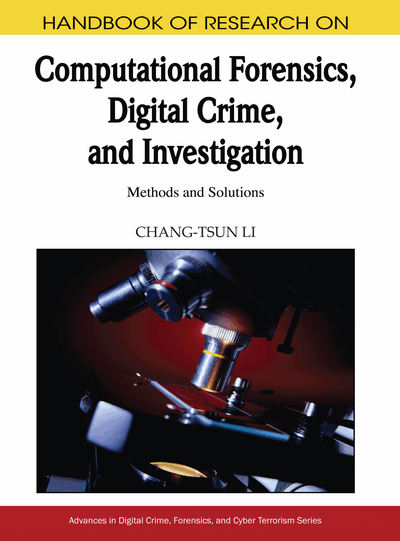# A Novel Multilevel DCT Based Reversible Data Hiding

Hong Cai (University of Texas at San Antonio, USA) and Sos S. Agaian (University of Texas at San Antonio, USA)
DOI: 10.4018/978-1-60566-836-9.ch009
Available
\$37.50
No Current Special Offers

## Abstract

DCT and wavelet based techniques have been widely used in image processing, for example, the applications involving JPEG, MPEG and JPEG2000. To combine the advantages of DCT and wavelet, we introduce in this chapter a novel multilevel DCT decomposition method by exploiting the modified inverse Hilbert curve. The experimental results showed that the proposed multilevel decomposition can extract characteristic DCT coefficients and assign the coefficients to new neighborhoods with distinct frequency properties. We discuss a powerful reversible data hiding algorithm in JPEG images based on this new multilevel DCT. This lossless data hiding algorithm features a key-dependent (multilevel structure) coefficient-extension technique and an embedding location selector, and it can achieve high quality reconstructed images with disparate content types.
Chapter Preview
Top

## 1. Introduction

Discrete Cosine Transform (DCT) and wavelet transform are widely used in image processing such as compression, recognition and information hiding. DCT, a sub-optimal transform, is favorably close to the optimal Karhunen-Loeve Transform (KLT). The popular JPEG image format adopts the DCT as the core transform technique owing to its low computation cost, good decorrelation and energy compaction properties (Rao et al., 1990). In the past decades, another efficient transform, the wavelet transform has been developed to exploit the multi-resolution property in signals. It was then successfully employed in the new image format standard known as JPEG 2000. The applications of DCT and wavelet transform span from signal compression, recognition, feature extraction (Ma et al., 2004; Chen et al., 2006; Jing et al., 2004; Guo et al., 2005), information retrieval, to clustering and biometrics (Zhang et al., 2004; Galvão et al., 2004; Kokare et al., 2005). Recently the multilevel DCT decomposition methods have been developed to combine the features of these two transforms. The success of these methods has been demonstrated in the fields of image compression and steganalysis (Xiong et al., 1996; Jeong et al., 1998; Agaian et al., 2005). However, two challenges remain present in multilevel DCT decomposition: firstly, how to efficiently exploit the position information of DCT coefficients, and secondly, how to extend this efficient decomposition to new application areas. In our previous work (Agaian et al., 2005), a template was utilized to rearrange DCT coefficients and to achieve multilevel decomposition. Then it was applied to detect hidden information.

In this chapter, we present a novel multilevel DCT decomposition method that use the modified inverse Hilbert curve (MIHC). A new reversible data hiding algorithm is developed based on this novel decomposition.

The first novelty of the present method is the introduction of the Hilbert curve, which takes position property into account. The Hilbert curve belongs to the family of space-filling curves, which has continuous mapping from a unit interval onto an N-dimensional unit cube where N < ∞ (Peano, 1890; Hilbert, 1891).

A Hilbert curve or Hilbert scanning order is considered as a scanning or a one-to-one mapping from a point in 2-dimensional (2D) space to a point in 1D sequence. The most important feature of the Hilbert curve is that it scans the proximate entry in 2D space continuously and then this scanning order preserves the point neighborhood properties (Peano, 1890; Hilbert, 1891;Gotsman et al., 1996).

## Key Terms in this Chapter

DCT: Discrete Cosine Transform (DCT) is a sum of cosine functions at different frequencies.

Wavelet: Mathematical functions that cut up data into different frequency components, and then study each component with a resolution matched to its scale.

Wavelet Structure: A multi level structure derived from wavelet coefficients at a given scale.

Reversible Data Hiding: A two-step data hiding procedure: first, embed (hide) data inside a media file and second, the hidden data and the original medial can be losslessly extracted.

JPEG Image: An image format is mostly common used by digital cameras, camcorder and other digital devices to store and transmit images.

Hilbert Curve: A kind of space-filling curve defined by David Hilbert in 1891.

Pure Payload: Actual data embedding capacity

## Complete Chapter List

Search this Book:
Reset